Function Repository Resource:

# NaturalEquations

Compute the natural equations of a curve

Contributed by: Wolfram Staff (original content by Eric W. Weisstein)
 ResourceFunction["NaturalEquations"][c,t] computes the natural equations of a curve c with parameter t.

## Details and Options

A natural equation is an equation that specifies a curve independent of any choice of coordinates or parametrization.
Natural equations are computed using the tangential angle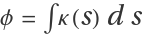, where κ is the curvature, s is the arc length, and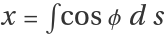and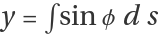are the coordinates.
ResourceFunction["NaturalEquations"] accepts the same options as Integrate.

## Examples

### Basic Examples (3)

Natural equations for a circle:

 In:=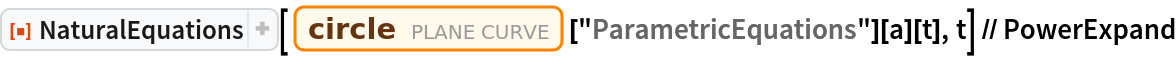Out=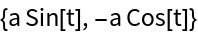Natural equations for several curves:

 In:=Out=Natural equations for some curves can be solved in terms of elementary functions. Get the equation for a logarithmic spiral:

 In:=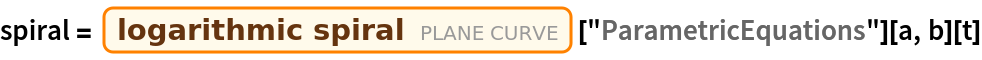Out=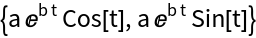Get the natural equations:

 In:=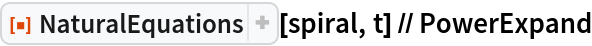Out=### Options (1)

#### Assumptions (1)

Use the option Assumptions to specify assumptions:

 In:=Out=### Properties and Relations (2)

The Cornu spiral:

 In:=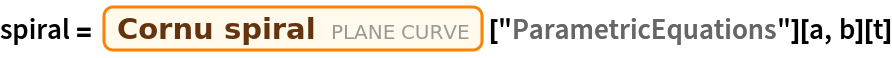Out=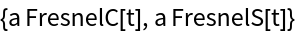NaturalEquations gives the same result:

 In:=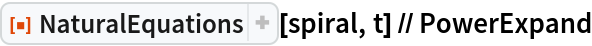Out=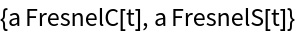Alfred Gray’s generalization of the Cornu spiral:

 In:=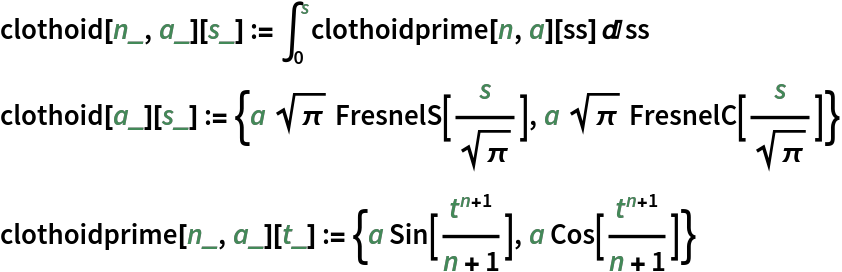In this case, the resulting natural equations contain special functions:

 In:=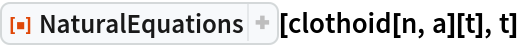Out=Using assumptions can simplify the resulting expressions:

 In:=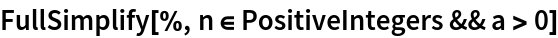Out=Get equations for the clothoid prime curve (the velocity of the nth clothoid):

 In:=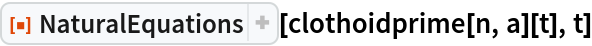Out=Simplifying:

 In:=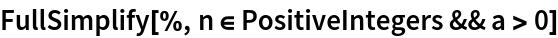Out=### Possible Issues (2)

Some cases can give large results:

 In:=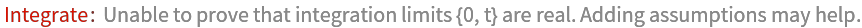Out=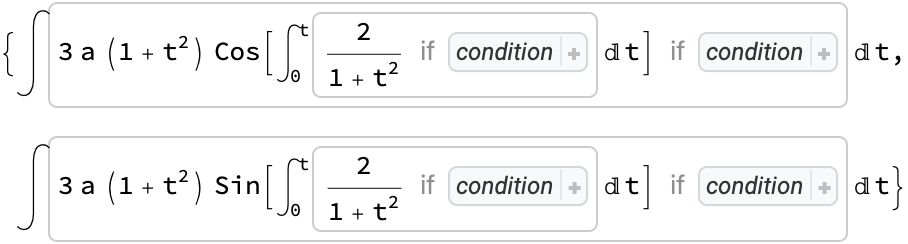But they can often be simplified:

 In:=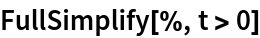Out=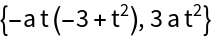Cassini curve:

 In:=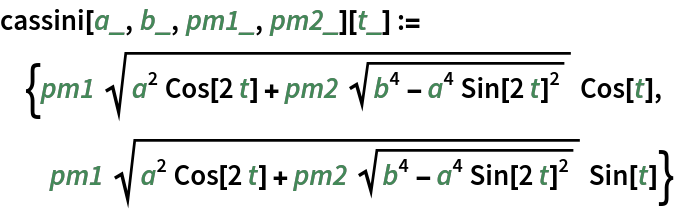Some cases need some time to evaluate:

 In:=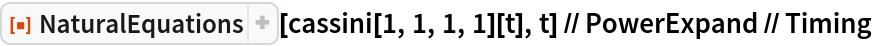Out=Enrique Zeleny

## Version History

• 1.0.0 – 12 June 2020